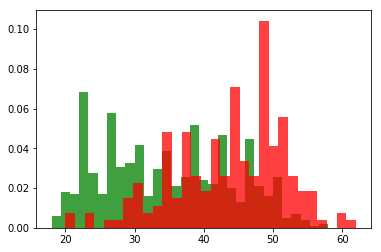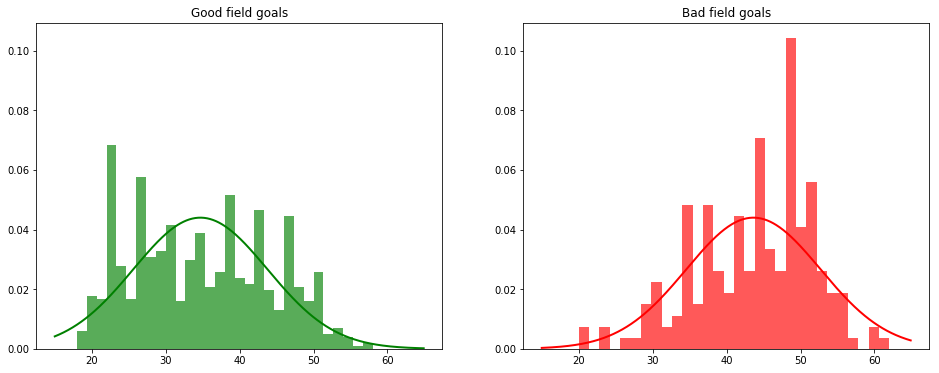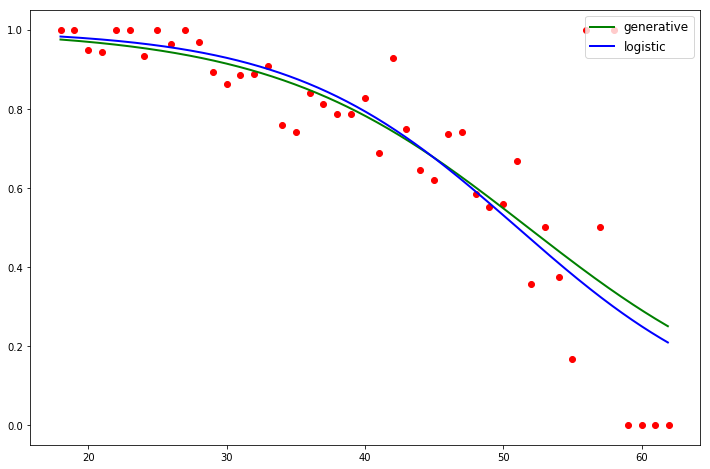This article is my notes on generative model for Lecture 5 and 6 of Machine Learning by Andrew Ng. What we do in logistic regression using generalized linear model is that, we approximate $P(y|x)$ using given data. This kind of learning algorithms is discriminative, in which we predict $y$ based on the input features $x$. On the contrary, generative model is to model $P(x|y)$, the probability of the features $x$ given class $y$. In other words, we want to study how the features structure looks like given a class $y$. If we also learn what $P(y)$ is, we can easily recover $P(y|x)$, for example, in the binary classification problem,

$$\begin{equation} P(y=1|x) = \frac{P(x|y=1)P(y=1)}{P(x)}, \label{eqn:bayes} \end{equation}$$

where $P(x) = P(x|y=0)P(y=0) + P(x|y=1)P(y=1)$.

In this article, we are going to see a simple example of generative model on Gaussian discriminant analysis and Naive Bayes.

## Gaussian Discriminant Analysis

Assume $x \in \mathbb{R}^n$. In Gaussian discriminant analysis, we assume $P(x|y)$ is Gaussian, i.e.,

$$P(x|y) = \frac{1}{(2\pi)^{\frac{n}{2}}|\Sigma|^{\frac{1}{2}}} \exp\left(-\frac{1}{2}(x-\mu)^T \Sigma^{-1} (x-\mu)\right),$$

where $\mu$ is the mean and $\Sigma$ is the covariance matrix.

Let's apply Gaussian discriminant anlysis to binary classifiaction problems, to get a generative model for prediction. In this model, we have

$$\begin{split} & P(y) = \phi^y (1-\phi)^{1-y} \\ & P(x|y=0) = \frac{1}{(2\pi)^{\frac{n}{2}}|\Sigma|^{\frac{1}{2}}} \exp\left(-\frac{1}{2}(x-\mu_0)^T \Sigma^{-1} (x-\mu_0)\right) \\ & P(x|y=1) = \frac{1}{(2\pi)^{\frac{n}{2}}|\Sigma|^{\frac{1}{2}}} \exp\left(-\frac{1}{2}(x-\mu_1)^T \Sigma^{-1} (x-\mu_1)\right) \end{split},$$

where $\phi, \mu_0, \mu_1$ and $\Sigma$ are parameters for which the log-likelihood function

$$\begin{split} l(\phi, \mu_0, \mu_1, \Sigma) &= \log \prod_{i=1}^m P(x^{(i)}, y^{(i)}) \\ &= \log \prod_{i=1}^m P(x^{(i)}|y^{(i)})P(y^{(i)}) \end{split}$$

is maximized. Here $P(x^{(i)}, y^{(i)})$ is the joint probability and $\{(x^{(i)}, y^{(i)})\}_{i=1}^m$ is the set of sample points.

When solved the maximization problem of $l(\phi, \mu_0, \mu_1, \Sigma)$, we get the parameters for the generative model:

$$\begin{split} & \phi = \frac{\sum_{i=1}^m y^{(i)}}{m} \\ & \mu_0 = \frac{\sum_{y^{(i)}=0}x^{(i)}}{\sum_{y^{(i)}=0}1} \\ & \mu_1 = \frac{\sum_{y^{(i)}=1}x^{(i)}}{\sum_{y^{(i)}=1}1} \\ & \Sigma = \frac{1}{m} \sum_{i=1}^m \left(x^{(i)}-\mu_{y^{(i)}}\right)\left(x^{(i)}-\mu_{y^{(i)}}\right)^T \end{split}$$

Once all parameters are known, we can then predict $y$ based on a given $x$.

$$y = \mathrm{argmax}_y P(y|x) = \mathrm{argmax}_y \frac{P(x|y)P(y)}{P(x)} = \mathrm{argmax}_y P(x|y)P(y).$$

In my previous article Generalized Linear Model(Examples), I built a logistic regression for good or bad field goals with yardage as the only feature. Now, I am going to use the above generative model to predict $P(y|x)$. In this simple example, $n=1$, so

$$\begin{equation} \begin{split} & P(x|y=0) = \frac{1}{\sqrt{2\pi \Sigma}} \exp\left(-\frac{(x-\mu_0)^2}{2\Sigma}\right) \\ & P(x|y=1) = \frac{1}{\sqrt{2\pi \Sigma}} \exp\left(-\frac{(x-\mu_1)^2}{2\Sigma}\right) \end{split} \label{eqn:simple} \end{equation}$$
import requests
import re
import matplotlib.pyplot as plt
import numpy as np

# retrive data online
fieldgoal_url = 'http://www.stat.ufl.edu/~winner/data/fieldgoal.dat'
response = requests.get(fieldgoal_url)
# extract sample points
data_pat = '(\d+)'
data = map(int, re.findall(data_pat, response.content))
x, y = data[::3], data[1::3]
# size of the sample set
m = len(x)

# seperate x based on whether the field goal is good or not
good_x = [x[i] for i in range(m) if y[i]==1]
bad_x = [x[i] for i in range(m) if y[i]==0]
plt.hist(good_x, 30, normed=30, color='green', alpha=0.75)
plt.show()# parameters
phi = len(good_x) / float(m)
mu = [0, 0]
mu = sum(good_x) / float(len(good_x))
sigma = sum((x[i]-mu[y[i]]) ** 2 for i in range(m)) / float(m)
print(phi, mu, mu, sigma)

(0.7974683544303798, 43.630208333333336, 34.69444444444444, 82.28603529360076)


Gaussian discriminant analysis assumes that in each class, the features $x$ are normal distributed. Below is a piece of codes to show the distributions of features.

from scipy.stats import norm

ax = plt.subplot(1, 2, 1)
plt.title('Good field goals')
plt.hist(good_x, 30, normed=30, color='green', alpha=0.65)
u = np.arange(15, 65, 0.1)
v = map(lambda t: norm.pdf(t, mu, np.sqrt(sigma)), u)
plt.plot(u, v, 'g', linewidth=2)

plt.subplot(1, 2, 2, sharey=ax)
u = np.arange(15, 65, 0.1)
v = map(lambda t: norm.pdf(t, mu, np.sqrt(sigma)), u)
plt.plot(u, v, 'r', linewidth=2)

fig = plt.gcf()
fig.set_size_inches(16, 6)
plt.show()We see from the above figure that features $x$ roughly fit the Gaussian distributions, but not the well. In order to compare this model to previous logistic regression, let's calculate $P(y=1|x)$ from the parameters. By Equation ($\ref{eqn:bayes}$),

$$\begin{equation} \begin{split} P(y=1|x) &= \frac{P(x|y=1)P(y=1)}{P(x)} \\ &= \frac{\phi P(x|y=1)}{\phi P(x|y=1) + (1-\phi)P(x|y=0)} \end{split} \label{eqn:prediction} \end{equation}$$

Recall that in the previous logistic regression, we used gradient ascent to obtain

(a, b) = (-0.12228007960331008, 6.237420310313901)

And logistic regression gives us a prediction:

$$h_{a, b}(x) = \frac{1}{1+e^{-ax-b}}.$$
# generative(x) = P(y=1|x)
def generative(x):
good = norm.pdf(x, mu, np.sqrt(sigma))

# logistic(x) is the prediction from logistic progression
a, b = (-0.12228007960331008, 6.237420310313901)
def logistic(x, a, b):
eta = a*x + b
return 1.0/(1 + np.exp(-eta))

# calculate the observed probability
import collections
gxc = collections.Counter(good_x)
min_x = min(x)
max_x = max(x)

observation = []
for i in range(min_x, max_x+1):
total = gxc[i] + bxc[i]
total = 1.0 if total == 0 else float(total)
observation.append(gxc[i]/total)

# plot observation
plt.plot(range(min_x, max_x+1), observation, 'ro')

# plot prediction from generative model
u = np.arange(min_x, max_x, 0.1)
v = map(generative, u)
plt.plot(u, v, 'g', linewidth=2, label='generative')

# plot prediction from logistic regression
v = map(lambda t: logistic(t, a, b), u)
plt.plot(u, v, 'b', linewidth=2, label='logistic')

fig = plt.gcf()
fig.set_size_inches(12, 8)
plt.legend(loc='upper right', prop={'size': 12})
plt.show()These two method give a very similar prediction! Why is that? Let's come back to Equation ($\ref{eqn:prediction}$).

$$\begin{split} P(y=1|x) &= \frac{\phi P(x|y=1)}{\phi P(x|y=1) + (1-\phi)P(x|y=0)} \\ &= \frac{1}{1+\frac{1-\phi}{\phi} \cdot \frac{P(x|y=0)}{P(x|y=1)}} \end{split}$$

And now put in the formulas ($\ref{eqn:simple}$) for $P(x|y=0)$ and $P(x|y=1)$,

$$\begin{split} \frac{P(x|y=0)}{P(x|y=1)} &= \exp\left(-\frac{(x-\mu_0)^2}{2\Sigma}+\frac{(x-\mu_1)^2}{2\Sigma}\right) \\ &= \exp\left(-\frac{\mu_1-\mu_0}{\Sigma} \cdot x - \frac{\mu_0^2-\mu_1^2}{2\Sigma}\right) \end{split}$$

Therefore,

$$\begin{split} P(y=1|x) &= \frac{1}{1+\frac{1-\phi}{\phi} \cdot \exp\left(-\frac{\mu_1-\mu_0}{\Sigma} \cdot x - \frac{\mu_0^2-\mu_1^2}{2\Sigma}\right)} \\ &= \frac{1}{1+ \exp\left(-\frac{\mu_1-\mu_0}{\Sigma} \cdot x - \frac{\mu_0^2-\mu_1^2}{2\Sigma} + \log \frac{1-\phi}{\phi}\right)} \end{split}$$

Indeed Gaussian discriminant analysis will imply logistic regression. We can then put the parameters from Gaussian discriminant analysis in the form of logistic regression.

A = (mu - mu) / sigma
B = (mu**2 - mu**2) / (2*sigma) - np.log((1-phi)/phi)
print('Parameters from Gaussian discriminant analysis:\n{}'.format((A, B)))

Parameters from Gaussian discriminant analysis:
(-0.10859392917650769, 5.6233369024140298)
(-0.12228007960331008, 6.237420310313901)

gau_abs_err = gra_abs_err = 0
for i, t in enumerate(range(min_x, max_x)):
gau_abs_err += abs(logistic(t, A, B)-observation[i])
gra_abs_err += abs(logistic(t, a, b)-observation[i])
print('Absolute error of Gaussian discriminant analysis: {}'.format(gau_abs_err))
print('Absolute error of gradien ascent: {}'.format(gra_abs_err))

Absolute error of Gaussian discriminant analysis: 4.41501707618
Absolute error of gradien ascent: 4.42931961272


In this example, Gaussian discriminant analysis is a little bit better than the logisitic regression from gradient ascent. Another advantage of Gaussian discriminant analysis, or in general that of generative analysis, is that we don't need many sample points to get a good prediction. This is because we have strong assumptions on the structure of the features.

We see from above calculation that Gaussian discriminant analysis implies logistic regression. A similar calculation can show that any exponential family of distributions will aslo imply logistic regression.

## Naive Bayes

In a generative model, we would like to model $P(x|y)$. Suppose that there are $n$ features $x = (x_1, x_2, ..., x_n)$, then the Naive Bayes assumption simply assume that all $x_i$ are conditionally independent. Therefore,

$$\begin{split} P(x|y) &= P(x_1, \dots, x_n|y) \\ &= P(x_1|y) \cdot P(x_2|x_1, y) \cdots P(x_n|x_1, \dots, x_{n-1}, y) \\ &= \prod_{i=1}^n P(x_i|y), \end{split}$$

where the second equality comes from chain rule of probability, and the last equality comes from the assumption that all $x_i$ are conditionally independent.

### Spam filter

Suppose we want to make a spam filter so that given an email, it can decide whether or not the email is a spam. To start with, we need a dictionary of words as features. For example, given a training set of emails, we can take all words that appear as the list of features. Suppose the list of words are ${w_1, w_2, \dots, w_n}$, and for each email, we convert it into a $n \times 1$ vector

$$x = \left[\begin{array}{c}x_1 \\ x_2 \\ \vdots \\ x_n\end{array}\right],$$

where $x_i \in \{0, 1\}$ indicates whether word $w_i$ appears in the email. After conversion, the training set becomes

$$\{(x^{(1)}, y^{(1)}), \dots, (x^{(m)}, y^{(m)})\}.$$

We impose naive Bayes assumption on the features $x$. In reality, this is not a correct assumption. For example, if the word "reminder" appears in the email, most likely, the word "tomorrow" will also appear in it. Even though naive Bayes assumption neglects these obvious dependence, it can provide a pretty good spam filter.

With navie Bayes assumption,

$$P(x|y) = \prod_{i=1}^{n} P(x_i|y),$$

so the parameters needed for the models are

$$\begin{split} & \phi_{j|y=1} = P(x_j=1|y=1) \\ & \phi_{j|y=0} = P(x_j=1|y=0) \\ & \phi_y = P(y=1) \end{split},$$

for $j = 1, 2, \dots, n$. These parameters will be determined by maximizing the joint likelihood function

$$L(\phi_y, \phi_{i|y=1}, \phi_{i|y=0}) = \prod_{i=1}^m P(x^{(i)}, y^{(i)}).$$

The maximum estimate of the joint likelihood function gives

$$\begin{equation} \begin{split} & \phi_{j|y=1} = \frac{\sum_{i=1}^m 1\{x^{(i)}_j=1, y^{(i)}=1\}}{\sum_{i=1}^m 1\{y^{(i)}=1\}} \\ & \phi_{j|y=0} = \frac{\sum_{i=1}^m 1\{x^{(i)}_j=1, y^{(i)}=0\}}{\sum_{i=1}^m 1\{y^{(i)}=0\}} \\ & \phi_y = \frac{\sum_{i=1}^m 1\{y^{(i)}=1\}}{m} \end{split} \label{eqn:naive_parameters} \end{equation}$$

Here $1\{\mathrm{proposition}\}$ is an indicator function which values $1$ if the proposition is true and $0$ otherwise.

Once all parameters are known, we can calculate $P(y=1|x)$ using Bayes rule as before

$$\begin{split} P(y=1|x) &= \frac{P(x|y=1)P(y=1)}{P(x)} \\ &= \frac{P(x|y=1)P(y=1)}{P(x|y=1)P(y=1)+P(x|y=0)P(y=0)} \\ &= \frac{P(y=1)\prod_{j=1}^n P(x_j|y=1)}{P(y=1)\prod_{j=1}^n P(x_j|y=1)+P(y=0)\prod_{j=1}^n P(x_j|y=0)}. \end{split}$$

However, here is a problem with the model. Suppose the k-th word $w_k$ never appears in the training set of emails, then based on the formula ($\ref{eqn:naive_parameters}$),

$$\begin{split} & P(x_k=1|y=1) = \phi_{k|y=1} = 0 \\ & P(x_k=1|y=0) = \phi_{k|y=0} = 0 \end{split}$$

Then when we try to use this model to predict whether an email containing the word $w_k$ is a spam, a '0/0' error will be encoutered. To solve this issue, we can apply Laplace smoothing to get

$$\begin{split} & \phi_{j|y=1} = \frac{\sum_{i=1}^m 1\{x^{(i)}_j=1, y^{(i)}=1\}+1}{\sum_{i=1}^m 1\{y^{(i)}=1\}+2} \\ & \phi_{j|y=0} = \frac{\sum_{i=1}^m 1\{x^{(i)}_j=1, y^{(i)}=0\}+1}{\sum_{i=1}^m 1\{y^{(i)}=0\}+2} \\ & \phi_y = \frac{\sum_{i=1}^m 1\{y^{(i)}=1\}}{m} \end{split}$$

### One variation

In the above model for spam filter, feature $x_i \in \{0, 1\}$ captures whether or not the word $w_i$ appears in the email. We can easily generalize this model to other data sets, with feature $x_i$ taking values in $\{1, 2, \dots, k\}$. The only difference is that instead of Bernoulli distribution, we now have to use multinomial distribution for $P(x_i|y)$.

### another variation

In the spam filter above, feature $x$ only captures whether or not words in the list appear in the emails. It ignores the numbers of times of words appearing in the emails. We are going to introduce another model which respects the numbers of times of words in the emails. For a given email with $l$ words, we let

$$x = (x_1, x_2, \dots, x_l)$$

to be the features of this email, where $x_i$ represents the index in the dictionaly of the i-th word in the email. That is to say, $w_{x_i}$ is the i-th word in the email.

The joint distribution in this model is

$$P(x, y) = P(y) \prod_{i=1}^l P(x_i|y).$$

Warning: the lenght $l$ for each email varies!

The parameters for the models are

$$\begin{split} & \phi_{k|y=1} = P(x_j=k|y=1) \\ & \phi_{k|y=0} = P(x_j=k|y=0) \\ & \phi_{y} = P(y=1) \end{split}$$

where $k=1, 2, \dots, n$ and $P(x_j=k)$ is the probability of the j-th word of the email being $w_k$. The likelihood function in the model is

$$L(\phi_{k|y=1}, \phi_{k|y=0}, \phi_y) = \prod_{i=1}^m \prod_{j=1}^{l_i} P(x^{(i)}_j|y^{(i)}),$$

where $l_i$ here is the length of the i-th email.

Given a training set, maximum likelihood estimate and Laplace smoothing together will give us

$$\begin{split} & \phi_{k|y=1} = \frac{\sum_{i=1}^m 1\{y^{(i)}=1\}\sum_{j=1}^{l_i} 1\{x^{(i)}_j=k\} + 1}{\sum_{i=1}^m 1\{y^{(i)}=1\}l_i + n} \\ & \phi_{k|y=0} = \frac{\sum_{i=1}^m 1\{y^{(i)}=0\}\sum_{j=1}^{l_i} 1\{x^{(i)}_j=k\} + 1}{\sum_{i=1}^m 1\{y^{(i)}=0\}l_i + n} \\ & \phi_y = \frac{\sum_{i=1}^m 1\{y^{(i)}=1\}}{m} \end{split}$$

This model is very suitable for textures data.